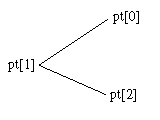﻿ L_AnnSetPoints | Function References | Raster Imaging C API Help
In This Topic ▼

# L_AnnSetPoints

#include "l_bitmap.h"

L_LTANN_API L_INT L_AnnSetPoints(hObject, pPoints, uCount)

Defines the shape of an annotation object, such as a polygon, that requires multiple points.

## Parameters

### HANNOBJECT hObject

Handle to the annotation object.

### pANNPOINT pPoints

Pointer to the array of LEADTOOLS ANNPOINT structures that specifies the shape of the annotation.

The ANNPOINT structure is like a Windows POINT structure, except that it uses double-precision floating point values.

Coordinates of an object's points are relative to its container object. The coordinates are interpreted using the container's scaling factors and offsets, which are described in Low-Level Coordinate System for Annotations.

### L_UINT uCount

The number of points in the array that you specify in the `pPoints` parameter.

#### Returns

Value Meaning
SUCCESS The function was successful.
< 1 An error occurred. Refer to Return Codes.

You call this function once, passing an array of points, which must be in the order in which the vertices are connected. To define the line that closes a polygon, the last point in the array is connected to the first point of the array.

Alternatively, you can use the L_AnnDefine function to specify the array of points interactively.

L_AnnSetPoints works only with the following types of objects:

• ANNOBJECT_POINTER
• ANNOBJECT_FREEHAND
• ANNOBJECT_LINE
• ANNOBJECT_POLYGON
• ANNOBJECT_POLYLINE
• ANNOBJECT_POINT
• ANNOBJECT_PROTRACTOR
• ANNOBJECT_FREEHANDHOTSPOT
• ANNOBJECT_TEXTPOINTER
• ANNOBJECT_POLYRULER

For annotation objects that are defined by a rectangle, use the L_AnnSetRect function.

You can position a Point object using the following:

````ANNPOINT apt; `
`L_AnnSetPoints(hPoint, apt, 1); ````

where apt contains the desired position.

To set the points of a cross-product object, do the following:

````ANNPOINT apt; `
`L_AnnGetPoints(hPoint, apt, 5); ````

hPoint is the handle to the cross-product object. apt and apt are the points for the primary ruler. apt is the intersection point. apt and apt are the points for the secondary ruler.

To set the points of a protractor object, do the following:

````ANNPOINT apt ; `
`L_AnnSetPoints(hPoint, apt, 3); ````

hPoint is the handle to the protractor object. apt and apt are the endpoints for the two rulers. apt is the intersection point. This is shown below:When calling L_AnnSetPoints on a ANNOBJECT_TEXTPOINTER object, specify three points. The three points are (Upper Left), (Lower Right), (Anchor).

Note: You can not create a line of two points which they are the same.

Required DLLs and Libraries

Platforms

Win32, x64.